Next: Relative velocity Up: Motion in 3 dimensions Previous: Motion with constant acceleration

## Projectile motion

As a simple illustration of the concepts introduced in the previous subsections, let us examine the following problem. Suppose that a projectile is launched upward from ground level, with speed, making an anglewith the horizontal. Neglecting the effect of air resistance, what is the subsequent trajectory of the projectile?

Our first task is to set up a suitable Cartesian coordinate system. A convenient system is illustrated in Fig. 16. The-axis points vertically upwards (this is a standard convention), whereas the-axis points along the projectile's initial direction of horizontal motion. Furthermore, the origin of our coordinate system corresponds to the launch point. Thus,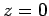corresponds to ground level.

Neglecting air resistance, the projectile is subject to a constant acceleration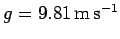, due to gravity, which is directed vertically downwards. Thus, the projectile's vector acceleration is written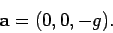(70)

Here, the minus sign indicates that the acceleration is in the minus-direction (i.e., downwards), as opposed to the plus-direction (i.e., upwards).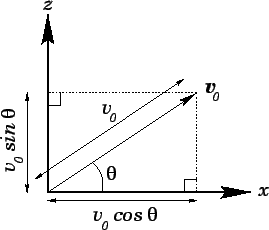What is the initial vector velocity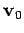with which the projectile is launched into the air at (say)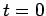? As illustrated in Fig. 16, given that the magnitude of this velocity is, its horizontal component is directed along the-axis, and its direction subtends an anglewith this axis, the components oftake the form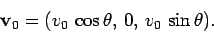(71)

Note thathas zero component along the-axis, which points into the paper in Fig. 16.

Since the projectile moves with constant acceleration, its vector displacement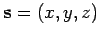from its launch point satisfies [see Eq. (64)]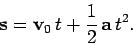(72)

Making use of Eqs. (70) and (71), the-,-, and-components of the above equation are written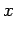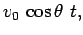(73)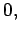(74)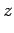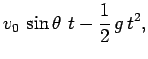(75)

respectively. Note that the projectile moves with constant velocity,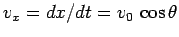, in the-direction (i.e., horizontally). This is hardly surprising, since there is zero component of the projectile's acceleration along the-axis. Note, further, that since there is zero component of the projectile's acceleration along the-axis, and the projectile's initial velocity also has zero component along this axis, the projectile never moves in the-direction. In other words, the projectile's trajectory is 2-dimensional, lying entirely within the-plane. Note, finally, that the projectile's vertical motion is entirely decoupled from its horizontal motion. In other words, the projectile's vertical motion is identical to that of a second projectile launched vertically upwards, at, with the initial velocity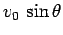(i.e., the initial vertical velocity component of the first projectile)--both projectiles will reach the same maximum altitude at the same time, and will subsequently strike the ground simultaneously.

Equations (73) and (75) can be rearranged to give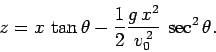(76)

As was first pointed out by Galileo, and is illustrated in Fig. 17, this is the equation of a parabola. The horizontal rangeof the projectile corresponds to its-coordinate when it strikes the ground (i.e., when). It follows from the above expression (neglecting the trivial result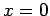) that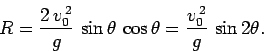(77)

Note that the range attains its maximum value,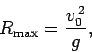(78)

when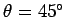. In other words, neglecting air resistance, a projectile travels furthest when it is launched into the air at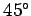to the horizontal.

The maximum altitude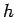of the projectile is attained when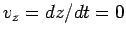(i.e., when the projectile has just stopped rising and is about to start falling). It follows from Eq. (75) that the maximum altitude occurs at time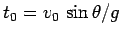. Hence,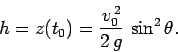(79)

Obviously, the largest value of,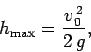(80)

is obtained when the projectile is launched vertically upwards (i.e.,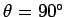).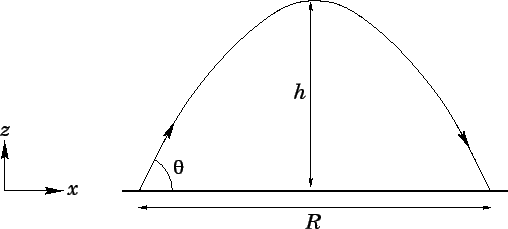Next: Relative velocity Up: Motion in 3 dimensions Previous: Motion with constant acceleration
Richard Fitzpatrick 2006-02-02